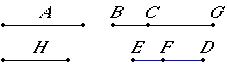# Proposition 88

To find the fourth apotome.

Set out a rational straight line A, and let BG be commensurable in length with it. Set out two numbers DF and FE such that the whole DE has to neither of the numbers DE nor EF the ratio which a square number has to a square number.

Let it be contrived that DE is to EF as the square on BG is to the square on GC. Then the square on BG is commensurable with the square on GC.

But the square on BG is rational, therefore the square on GC is also rational. Therefore GC is rational.X.9

Now, since DE does not have to EF the ratio which a square number has to a square number, therefore neither has the square on BG to the square on GC the ratio which a square number has to a square number. Therefore BG is incommensurable in length with GC.

X.73

And both are rational, therefore BG and GC are rational straight lines commensurable in square only. Therefore BC is an apotome.

Now let the square on H be that by which the square on BG is greater than the square on GC.

V.19,Cor.

Since DE is to EF as the square on BG is to the square on GC, therefore, in conversion, ED is to DF as the square on GB is to the square on H.

X.9

But ED does not have to DF the ratio which a square number has to a square number, therefore neither has the square on GB to the square on H the ratio which a square number has to a square number. Therefore BG is incommensurable in length with H.

And the square on BG is greater than the square on GC by the square on H, therefore the square on BG is greater than the square on GC by the square on a straight line incommensurable with BG.

And the whole BG is commensurable in length with the rational straight line A set out. Therefore BC is a fourth apotome.

X.Def.III.4

Therefore the fourth apotome has been found.

Q.E.F.

## Guide

This proposition is not used in the rest of the Elements.